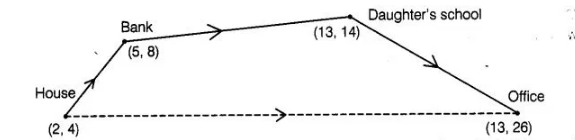# Ayush starts walking from his house to office.

Question:

Ayush starts walking from his house to office. Instead of going to the office directly, he goes to a bank first, from there to his daughter’s school and then

reaches the office. What is the extra distance travelled by Ayush in reaching his office? (Assume that all distance covered are in straight lines). If the

house is situated at (2, 4), bank at (5, 8), school at (13,14) and office at (13, 26) and coordinates are in km.

Solution:By given condition, we drawn a figure in which every place are indicated with his coordinates and direction also.

We know that,

distance between two points $\left(x_{1}, y_{1}\right)$ and $\left(x_{2}, y_{2}\right)$.

$d=\sqrt{\left(x_{2}-x_{1}\right)^{2}+\left(y_{2}-y_{1}\right)^{2}}$

Now, distance between house and bank $=\sqrt{(5-2)^{2}+(8-4)^{2}}$

$=\sqrt{(3)^{2}+(4)^{2}}=\sqrt{9+16}=\sqrt{25}=5$

Distance between bank and daughter's school

$=\sqrt{(13-5)^{2}+(14-8)^{2}}=\sqrt{(8)^{2}+(6)^{2}}$

$=\sqrt{64+36}=\sqrt{100}=10$

Distance between daughter's school and office $=\sqrt{(13-13)^{2}+(26-14)^{2}}$

$=\sqrt{0+(12)^{2}}=12$

Total distance (House + Bank + School + Office) travelled = $5+10+12=27$ units

Distance between house to offices $=\sqrt{(13-2)^{2}+(26-4)^{2}}$

$=\sqrt{(11)^{2}+(22)^{2}}=\sqrt{121+484}$

$=\sqrt{605}=24.59 \approx 24.6 \mathrm{~km}$

So, extra distance travelled by Ayush in reaching his office = 27 – 24.6 = 2.4 km Hence, the required extra distance travelled by Ayush is 2.4 km.